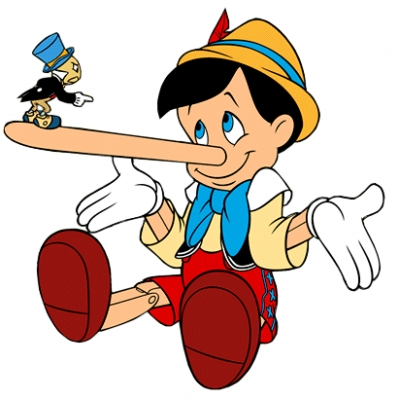The computer is like a truth machine: you can ask it something and it will tell you whether it is true or false.

Unlike Pinocchio, the computer never lies.# True or false?

When you write a statement to the computer, the computer answers you by `true` or `false`:

1. `true` means that your statement is true
2. `false` means that your statement is false

Today, we are going to learn about three kind of statements:

1. `=`: a statement that two things are equal
2. `>`: a statement that the first thing is greater than the second thing
3. `<`: a statement that the first thing is less than the second thing

For instance, it is true that `2` and `2` equals `4`:

``````(= (+ 2 2) 4)
``````

It is also true that `7` times `8` is `56`:

``````(= (* 7 8) 56)
``````

But it is false that `5` times `6` is `31`:

``````(= (* 5 6) 31)
``````

Can you modify the statement above and make it true?

It is true that `5` times `7` is greater than `10`:

``````(> (* 5 7) 10)
``````

But it is false that `2` times `2` is greater than `100`:

``````(> (* 2 2) 100)
``````

Can you modify the statement above and make it true?

# Exercises

A. Ask the computer if it is true that `7` times `6` equals `40`:

``````()
``````

You should get `false`.

B. Is it true that `5` and `7` is less than `13`?

``````()
``````

You should get `true`.

C. Is it true that `3` times `4` is `12`?

``````()
``````

You should get `true`.

D. Is it true that `3` times `6` equals `10` and `8`?

``````()
``````

You should get `true`.

E. Is it true that `7` times `7` is greater than `10` times `6`?

``````()
``````

You should get `false`.

Send us a screenshot with your programs to viebel@gmail.com.